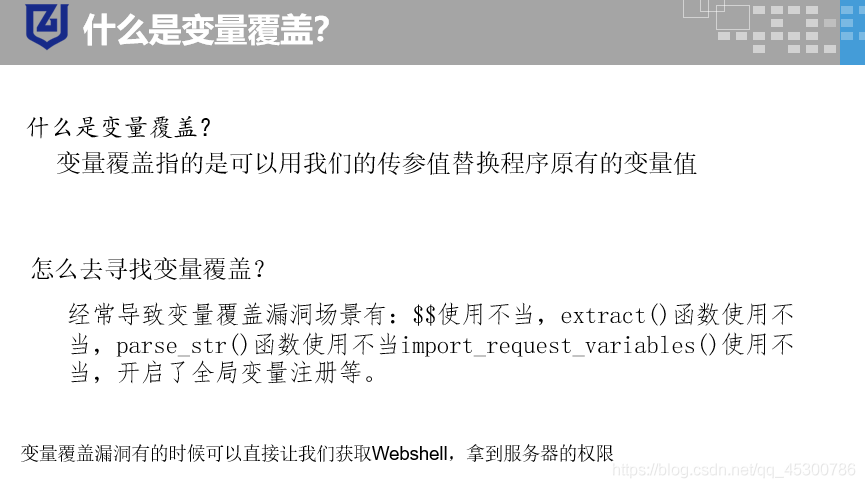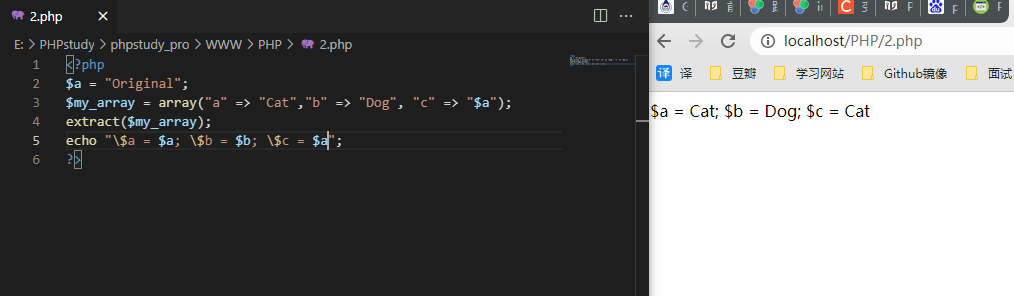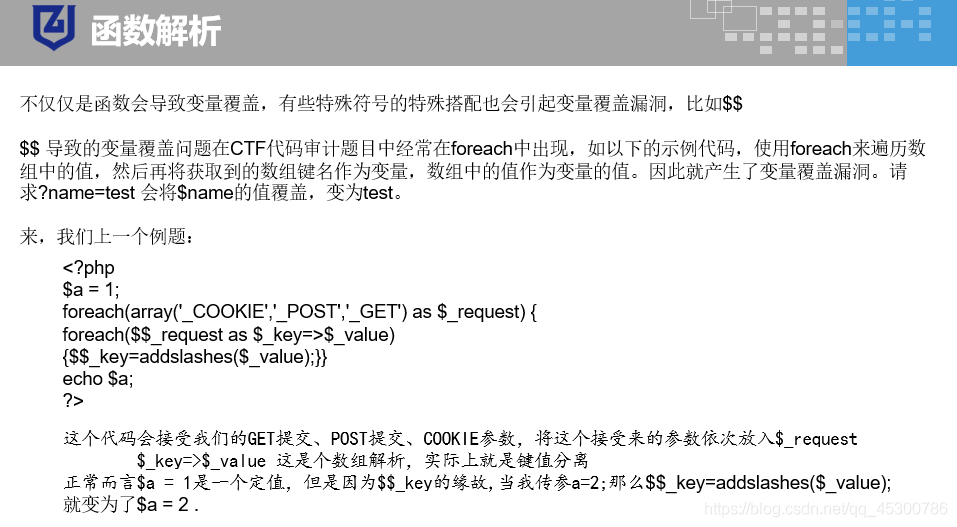#### 变量覆盖``````<?php        //ctf考题对flag过滤
\$a = fl;
\$b = ag;
\$c = \$a.\$b;
?>
----------
<?php        //对assert断言函数过滤
\$bb="assert";
\$aa='bb';
\$\$aa(\$_POST['a']);
?>
-----------
``````

##### extract

extract() 函数从数组中将变量导入到当前的符号表

``````<?php
\$a = "Original";
\$my_array = array("a" => "Cat","b" => "Dog", "c" => "Horse");
extract(\$my_array);
echo "\\$a = \$a; \\$b = \$b; \\$c = \$c";
?>
---------------------------

``````##### \$\$##### parse_str

``````<?php
\$a = 1;
parse_str("foo_bar=42",\$o);
print_r(\$o);

echo "<br>";

parse_str("%20news[id%00=42",\$o);  //Array ( [news_id] => 42 )
print_r(\$o);
//  %20news[id%00=42
?>
``````

##### import_request_variables

import_request_variables 函数可以在 register_global = off 时，把 GET/POST/Cookie 变量导入全局作用域中

``````<?php
\$a = '0';
import_request_variables('G');

if(\$a == 1){
echo "hello!";
}else{
echo "word!";
}
?>
``````